Posted on

# Card detectionMichael Garaj

The goal of this project is to detect card in captured image. Motivation was to make automatized recognizer of cards for poker tournaments. Application is implemented to find orthogonal edges in an image and try to find card by ratio of its edges.

Process of finding and recognizing a card in image follows these steps:

1. Load an image from local repository.
2. Apply blur and bilateral filter.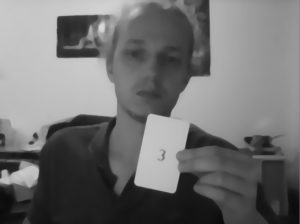3. Compute binary threshold.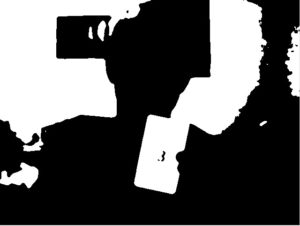4. Extract edges from binary image by Canny algorithm.
5. Apply Hough lines to get lines find in edge image.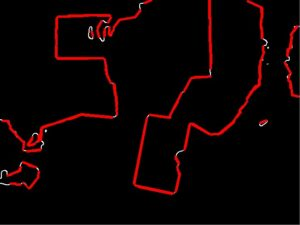6. Search for orthogonal lines and store them in structure for future optimalization.
7. Optimise number of detected lines in same area by choosing only the biggest ones.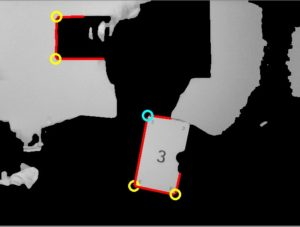8. Find card which consist of 3 touching lines.
9. Compute ratio of the lines and identify cards in the image.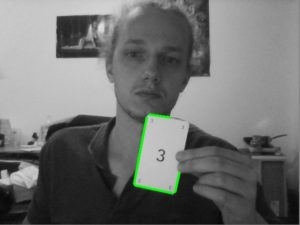Following code sample shows steps of optimalization of detected corners:

```vector<MyCorner> optimalize(vector<MyCorner> corners, Mat image) {
vector<MyCorner> optCorners;

for (int i = 0; i < corners.size(); i++) {
corners[i].crossing = crossLines(corners[i]);
corners[i].single = 1;
}

int distance = 25;
for (int i = 0; i < corners.size() - 1; i++) {
MyCorner corner = corners[i];
float lengthI = 0, lengthJ = 0;

if (corner.single){
for (int j = i + 1; j < corners.size(); j++) {

if (abs(corner.crossing.x - corners[j].crossing.x) < distance && abs(corner.crossing.y - corners[j].crossing.y) < distance &&
(corner.single || corners[j].single)) {

lengthI = getLength(corner.u) + getLength(corner.v);
lengthJ = getLength(corners[j].u) + getLength(corners[j].v);

if (lengthI < lengthJ) {
corner = corners[j];
}
corner.single = 0;
corners[i].single = 0;
corners[j].single = 0;
}
}
optCorners.push_back(corner);
}
}

return optCorners;
}
```